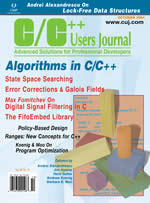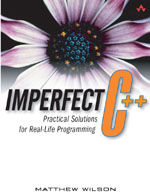# Boost Iterable Range Library

Welcome to the home of Boost Iterable Range Library, which provides information about the Iterable Range concept (there are three Range concepts: Notional Range, Iterable Range and Indirect Range; for more details, see RangeLib).

The Range concept is the result of a collaboration between John Torjo and Matthew Wilson, both frustrated by limitations in the STL Iterator concept. Among their other incarnations, John and Matthew are contributing editors to C/C++ Users Journal, in which the Range concept has been documented in recent articles.

## Contents

An Iterable Range is a smarter pair of iterators (begin and end). It allows manipulation of both iterators (begin and end), and preserves the underlying iterator type (for example, if the underlying iterators are bidirectional, so will the range be).

The fact that an iterable range holds two iterators (representing its position and its end position) makes it easier to:

• create (and possibly filter) sub-ranges
• implement range algorithms (all the STL algorithms have Iterable Range counterparts). The range algorithms are smart, and work for containers as well. Any container you pass to a range algorithm will automatically be converted to an Iterable Range.
• perform range composition (take a range as input, and yield another range as output)

## Basic usage

All of the Iterable Range definitions and operations follow modern techniques. They all reside in the boost::rangelib namespace.

When you construct a range, you either construct it from a container, or a pair of iterators. When you know the container type, use crange:

```typedef std::vector<int> array;
array a;
crange<array> r(a); // non-const range
crange<const array> const_r(a); // const range
```

Whe you know only the iterator type, use irange:

```template<class iterator> void print_range(iterator begin, iterator end) {
for ( irange<iterator> r(begin,end); r; ++r)
std::cout << *r << std::endl;
}
```

## Range algorithms

Because the fact that an Iterable Range contains two iterators is a prerequisite, adapting all STL algorithms to work for Iterable Ranges is quite simple. In order to use an STL algorithm adapted for Iterable Range, just use the rng:: prefix instead of std::

```  std::vector<int> v;
std::list<int>   l;

// print vector
rng::copy(v, std::ostream_iterator<int>(std::cout," "));
// copy vector to list
rng::copy(v, std::back_inserter(l));
```

## Range Composition

One of the most powerful features of the Iterable Range concept is "Range Composition". You can take one range as input, and yield another one as output (which you usually use in algorithms).

The simplest example is filtering - you can filter an existing range and/or container.

```  bool is_even(int i){ return (i % 2) == 0; }

...

std::list l;
// ... fill it
// print only even numbers
rng::copy(filtered(l, &is_even), std::ostream_iterator<int>(std::cout, " "));
```

The result of one composition can be the input for another composition. For instance, you can print the squared of each even number:

```// ... same as above
int square(int n) { return n * n }
rng::copy(transformed(filtered(l,&is_even),&sqare), std::ostream_iterator<int>(...));```

In order for range composition to work, we have provided the following range adaptors.

A range adaptor is a function that adapts an iterator in order to be used within an Iterable Range as simple as possible.

Consided the filtered example above. Had we not used an adaptor, we should have done something similar to:

```typedef boost::filter_iterator<std::list<int>::iterator> iterator;
irange<iterator> r( iterator(l.begin(),&is_even), iterator(l.end(),&is_even) );
rng::copy( r, std::ostream_iterator<int>(...) );```

Of course, in client code, and especially in generic code, this can become a maintenance nightmare (also, note that in real code, you won't use names like 'l', so the hush-mush code will get even worse). And what about when you want to do a double-composition (like the transformed filtered range example above)? It could become close to impossible to get it right the first time.

• filtered( range, predicate). Returns a range that contains only the elements int the original range that match the given predicate.
• transformed( range, func). For each element in the original range, applies func and returs the result.
• breaked( range, predicate). Returns the original range up-to the first time when predicate(*iterator) returns false. Similar to a manual-loop with an internal "if (!predicate(*iter)) break;"
• indirected( range). Dereferences each element in the range. Useful when you have a container of pointers, and want to manipulate the pointed values.
• resorted( range [,pred]). Returns the original range re-sorted, leaving the original range unchanged.
• reversed( range). Returns the original range reversed (from rbegin() to rend() ).
• generated( generator, stopper_pred). Creates a range by using a generator() function (similar to std::generate/ std::generate_n algorithms). After generating one element, the stopper_pred is asked if it should generate more elements. The range ends when stopper_pred returns false.

Finally, note that when you download the code, there are examples for each of the above adaptors.

## Related Publications

### Articles

•  "Ranges, part 2: Iterable Range Adaptors, Algorithms and Composition", John Torjo and Matthew Wilson, C/C++ User's Journal, Accepted, pending publication
•  "Ranges, part 1: Concepts and Implementation", Matthew Wilson and John Torjo, C/C++ User's Journal, Volume 22 Number 10, October 2004•  "Adapting Callback Enumeration APIs to the Input Iterator Concept", Matthew Wilson, C/C++ User's Journal, Volume 22 Number 2, February 2004

### Books

•  Ranges are also discussed in Chapter 34 of Matthew Wilson's new book Imperfect C++ (Addison-Wesley, Oct 2004)